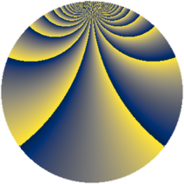# Properties

 Label 1520.2.djLevel $1520$ Weight $2$ Character orbit 1520.dj Rep. character $\chi_{1520}(79,\cdot)$ Character field $\Q(\zeta_{18})$ Dimension $360$ Sturm bound $480$

# Related objects

## Defining parameters

 Level: $$N$$ $$=$$ $$1520 = 2^{4} \cdot 5 \cdot 19$$ Weight: $$k$$ $$=$$ $$2$$ Character orbit: $$[\chi]$$ $$=$$ 1520.dj (of order $$18$$ and degree $$6$$) Character conductor: $$\operatorname{cond}(\chi)$$ $$=$$ $$380$$ Character field: $$\Q(\zeta_{18})$$ Sturm bound: $$480$$

## Dimensions

The following table gives the dimensions of various subspaces of $$M_{2}(1520, [\chi])$$.

Total New Old
Modular forms 1512 360 1152
Cusp forms 1368 360 1008
Eisenstein series 144 0 144

## Trace form

 $$360q + O(q^{10})$$ $$360q - 36q^{41} - 180q^{49} + 72q^{85} + 72q^{89} + O(q^{100})$$

## Decomposition of $$S_{2}^{\mathrm{new}}(1520, [\chi])$$ into newform subspaces

The newforms in this space have not yet been added to the LMFDB.

## Decomposition of $$S_{2}^{\mathrm{old}}(1520, [\chi])$$ into lower level spaces

$$S_{2}^{\mathrm{old}}(1520, [\chi]) \cong$$ $$S_{2}^{\mathrm{new}}(380, [\chi])$$$$^{\oplus 3}$$